# Hexacontagon

Regular hexacontagon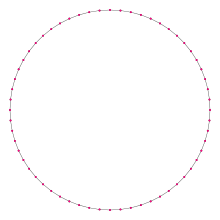A regular hexacontagon
Type Regular polygon
Edges and vertices 60
Schläfli symbol {60}, t{30}, tt{15}
Coxeter diagramSymmetry group Dihedral (D60), order 2×60
Internal angle (degrees) 174°
Dual polygon Self
Properties Convex, cyclic, equilateral, isogonal, isotoxal

In geometry, a hexacontagon or hexecontagon or 60-gon is a sixty-sided polygon. The sum of any hexacontagon's interior angles is 10440 degrees.

## Regular hexacontagon properties

A regular hexacontagon is represented by Schläfli symbol {60} and also can be constructed as a truncated triacontagon, t{30}, or a twice-truncated pentadecagon, tt{15}. A truncated hexacontagon, t{60}, is a 120-gon, {120}.

One interior angle in a regular hexacontagon is 174°, meaning that one exterior angle would be 6°.

The area of a regular hexacontagon is (with t = edge length)The circumradius of a regular hexacontagon isThis means that the trigonometric functions of π/60 can be expressed in radicals.

### Constructible

Since 60 = 22 × 3 × 5, a regular hexacontagon is constructible using a compass and straightedge. As a truncated triacontagon, it can be constructed by an edge-bisection of a regular triacontagon.

## Symmetry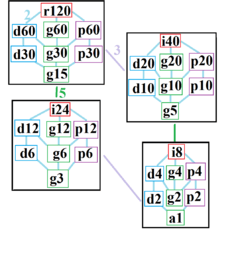The symmetries of a regular hexacontagon, divided into 4 subgraphs containing index 2 subgroups. Each symmetry within a subgraph is related to the lower connected subgraphs.

The regular hexacontagon has Dih60 dihedral symmetry, order 120, represented by 60 lines of reflection. Dih60 has 11 dihedral subgroups: (Dih30, Dih15), (Dih20, Dih10, Dih5), (Dih12, Dih6, Dih3), and (Dih4, Dih2, Dih1). And 12 more cyclic symmetries: (Z60, Z30, Z15), (Z20, Z10, Z5), (Z12, Z6, Z3), and (Z4, Z2, Z1), with Zn representing π/n radian rotational symmetry.

These 24 symmetries are related to 32 distinct symmetries on the hexacontagon. John Conway labels these lower symmetries with a letter and order of the symmetry follows the letter. He gives d (diagonal) with mirror lines through vertices, p with mirror lines through edges (perpendicular), i with mirror lines through both vertices and edges, and g for rotational symmetry. a1 labels no symmetry.

These lower symmetries allows degrees of freedom in defining irregular hexacontagons. Only the g60 symmetry has no degrees of freedom but can seen as directed edges.

## Hexacontagram

A hexacontagram is a 60-sided star polygon. There are 7 regular forms given by Schläfli symbols {60/7}, {60/11}, {60/13}, {60/17}, {60/19}, {60/23}, and {60/29}, as well as 22 compound star figures with the same vertex configuration.

 Picture Interior angle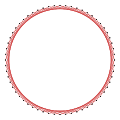{60/7}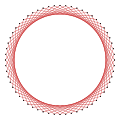{60/11}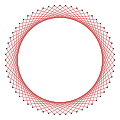{60/13}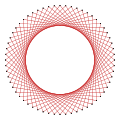{60/17}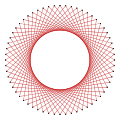{60/19}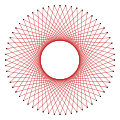{60/23}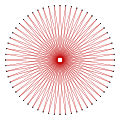{60/29} 138° 114° 102° 78° 66° 42° 6°

## References

1. Gorini, Catherine A. (2009), The Facts on File Geometry Handbook, Infobase Publishing, p. 78, ISBN 9781438109572.
2. Constructible Polygon
3. John H. Conway, Heidi Burgiel, Chaim Goodman-Strauss, (2008) The Symmetries of Things, ISBN 978-1-56881-220-5 (Chapter 20, Generalized Schaefli symbols, Types of symmetry of a polygon pp. 275-278)